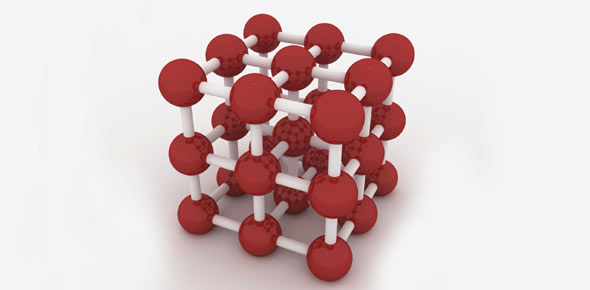# Chemistry Quiz 2 (Atoms)

10 Questions | Total Attempts: 318SettingsSubatomic particles

• 1.
The mass of the atom is located
• A.

In the nucleus

• B.

In the electron cloud

• C.

In the protons only

• D.

In the electrons only

• 2.
The nucleus contains (check all that apply)
• A.

Protons

• B.

Neutrons

• C.

Electrons

• 3.
How are all elements unique?
• A.

The mass number

• B.

The atomic number

• C.

Their appearance

• D.

Their state of matter

• 4.
An atom that is electrically stable will have the same number of protons and electrons.
• A.

True

• B.

False

• 5.
Which of the following is correct?
• A.

Protons are positive but have no mass.

• B.

Protons are neutral with a mass of 1.

• C.

Electrons are negative and have no mass.

• D.

Electrons are positive and have no mass.

• 6.
The element nitrogen has a mass of 14 and 7 protons and 7 electrons. How many neutrons?
• 7.
In order to figure out the mass, what two numbers are added?
• 8.
Jamie and Sally are working on their homework together. Jamie said that sodium must have 11.9 neutrons because the mass is 22.9 and the atomic number is 11. Sally explained that this is incorrect because
• A.

Neutrons are the same as protons

• B.

The mass must be rounded because you can not have 0.9 of a neutron.

• C.

The mass number is the same as the number of neutrons.

• D.

Sodium is not really an element since she can't find it on the periodic table.

• 9.
The correct term for the protons, neutrons and electrons is
• A.

Subatomic particles

• B.

Atomic parts

• C.

Elemental particles

• D.

Charged particles

• 10.
What is the difference between the mass number and the atomic number of an atom?
Related TopicsBack to top